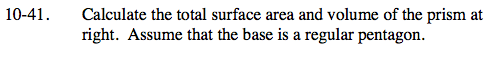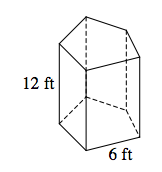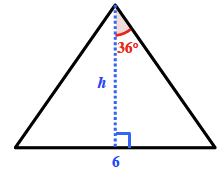### Home > GC > Chapter 10 > Lesson 10.1.4 > Problem10-41

10-41.Find the central angle of the pentagon.

$\frac{360^{\circ}}{5}=72^{\circ}$

$\text{tan}(36^{\circ})=\frac{3}{h} \ \ h=\frac{3}{\text{tan}(36^{\circ})} \ \ h \approx 4.1291 \text{ft}$

Area of the triangle = (0.5)(4.1291)(6) ≈ 12.39 ft²
Area of pentagon = (5)(12.39) ≈ 61.9 ft²

Find the area of each of the sides, and sum them to find the surface area.
(12)(6)(5) = 360
360 + 2(61.9) ≈ 483.8 ft²

Multiply the area of the pentagon by the height to find the volume.
(61.9)(12) ≈ 743.3 ft³The area of the triangle will help you find the area of the pentagon.Volume ≈ 743.3 ft³
Surface area ≈ 483.3 ft²